• 这是粒子群算法的一个文件，含有相关讲解和代码，代码复制到matlab中就可以用了
• 粒子群算法实例 matlab。 最近初学粒子群算法，自己按照自己的理解把每个步骤都解释了，然后每个步骤都解释的很清楚，很适合初学者观看，里面有matlab的代码，还有文档讲解，反正对于我一个初学者来说是能够看得懂得...
• 粒子群算法作为MATLAB智能算法之一，其优点是简单好用
• 粒子群算法是智能优化算法中的一个重要的组成部分，本代码是其在多目标领域一个重要的应用。看了此代码对多目标优化是及其重要的！
• 前面介绍了《粒子群算法》的基本原理，这里结合实例，看看粒子群算法是怎样解决实际问题的。采用过的函数和《遗传算法实例》中的一样： f(x)=x+10sin5x+7cos4xf(x) = x + 10\sin 5x + 7\cos 4x求其在区间[-10,10]...
前面介绍了《粒子群算法》的基本原理，这里结合实例，看看粒子群算法是怎样解决实际问题的。采用过的函数和《遗传算法实例》中的一样： f(x)=x+10sin5x+7cos4x<!--//--><![CDATA[//><!--
f(x) = x + 10\sin 5x + 7\cos 4x
//--><!]]>求其在区间[-10,10]之间的最大值。下面是该函数的图像：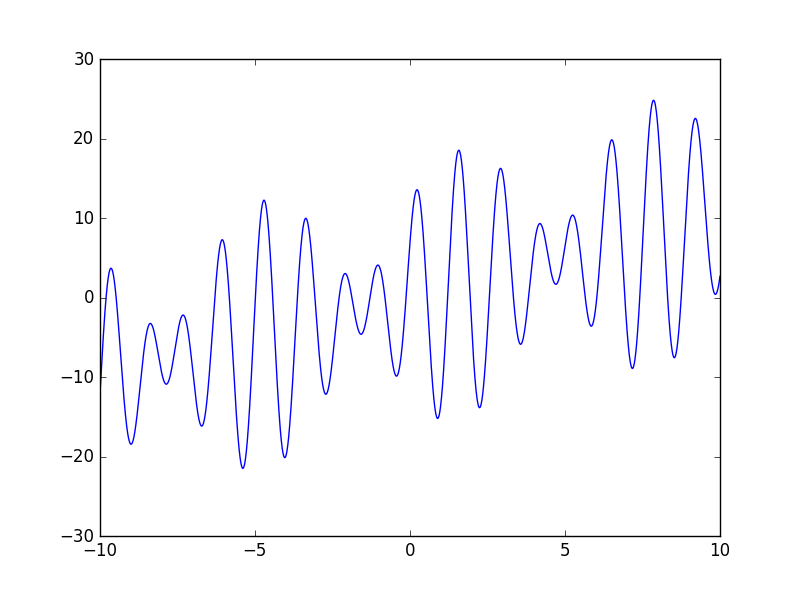在本例中，我们可以把x作为个体的染色体，函数值f(x)作为其适应度值，适应度越大，个体越优秀，最大的适应度就是我们要求的最大值。
直接看代码吧（直接看注释就能看懂）。

# -*- coding: utf-8 -*-

import numpy as np

# 粒子（鸟）
class particle:
def __init__(self):
self.pos = 0  # 粒子当前位置
self.speed = 0
self.pbest = 0  # 粒子历史最好位置

class PSO:
def __init__(self):
self.w = 0.5  # 惯性因子
self.c1 = 1  # 自我认知学习因子
self.c2 = 1  # 社会认知学习因子
self.gbest = 0  # 种群当前最好位置
self.N = 20  # 种群中粒子数量
self.POP = []  # 种群
self.iter_N = 100  # 迭代次数

# 适应度值计算函数
def fitness(self, x):
return x + 10 * np.sin(5 * x) + 7 * np.cos(4 * x)

# 找到全局最优解
def g_best(self, pop):
for bird in pop:
if bird.fitness > self.fitness(self.gbest):
self.gbest = bird.pos

# 初始化种群
def initPopulation(self, pop, N):
for i in range(N):
bird = particle()
bird.pos = np.random.uniform(-10, 10)
bird.fitness = self.fitness(bird.pos)
bird.pbest = bird.fitness
pop.append(bird)

# 找到种群中的最优位置
self.g_best(pop)

# 更新速度和位置
def update(self, pop):
for bird in pop:
# 速度更新
speed = self.w * bird.speed + self.c1 * np.random.random() * (
bird.pbest - bird.pos) + self.c2 * np.random.random() * (
self.gbest - bird.pos)

# 位置更新
pos = bird.pos + speed

if -10 < pos < 10: # 必须在搜索空间内
bird.pos = pos
bird.speed = speed
# 更新适应度
bird.fitness = self.fitness(bird.pos)

# 是否需要更新本粒子历史最好位置
if bird.fitness > self.fitness(bird.pbest):
bird.pbest = bird.pos

# 最终执行
def implement(self):
# 初始化种群
self.initPopulation(self.POP, self.N)

# 迭代
for i in range(self.iter_N):
# 更新速度和位置
self.update(self.POP)
# 更新种群中最好位置
self.g_best(self.POP)

pso = PSO()
pso.implement()

for ind in pso.POP:
print("x=", ind.pos, "f(x)=", ind.fitness)


某一次执行中生成的种群结果：
x= 7.44390041845   f(x)= 2.34326279816
x= 9.48378207609   f(x)= 13.3821268397
x= 6.3672261374    f(x)= 17.0548851104
x= 7.85674414126   f(x)= 24.855362869
x= 7.85674414216   f(x)= 24.855362869
x= 7.85674414201   f(x)= 24.855362869
x= 7.85674414185   f(x)= 24.855362869
x= 8.02319542929   f(x)= 20.1093330013
x= 7.85674414327   f(x)= 24.855362869
x= 7.85674414414   f(x)= 24.855362869
x= 7.85674414288   f(x)= 24.855362869
x= 7.85674414296   f(x)= 24.855362869
x= 7.85674414178   f(x)= 24.855362869
x= 7.85674414174   f(x)= 24.855362869
x= 7.8567441494    f(x)= 24.855362869
x= 6.44588532539   f(x)= 19.2820411821
x= 7.85674414041   f(x)= 24.855362869
x= 9.93067628809   f(x)= 1.12241685006
x= 7.8567441425    f(x)= 24.855362869
x= 8.81867117742   f(x)= 4.6512749143
得到的最优解结果为：
X=7.85674414174   f(x)=24.855362869
从图像上看符合要求。其结果图像如下，红色点表示种群中个体的位置。

# 绘图代码
import matplotlib.pyplot as plt
def func(x):
return x + 10 * np.sin(5 * x) + 7 * np.cos(4 * x)

x = np.linspace(-10, 10, 10000)
y = func(x)

scatter_x = np.array([ind.pos for ind in pso.POP])
scatter_y = np.array([ind.fitness for ind in pso.POP])
plt.plot(x, y)
plt.scatter(scatter_x, scatter_y, c='r')
plt.show()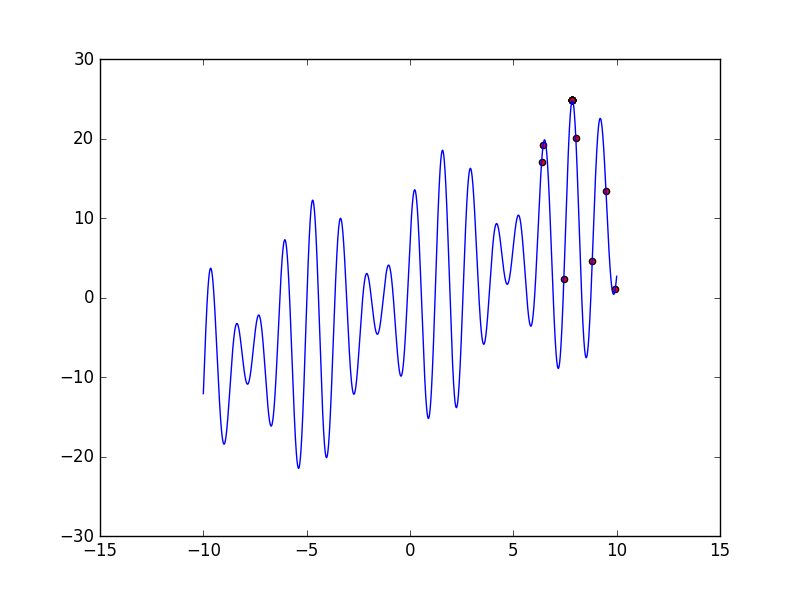展开全文• 粒子群算法求解配电网故障定位评价函数的极小值即可。 Matlab源代码：  clc;  clear all;  global Ij;  global Vmax; %允许误差  MaxNum=120; %粒子最大迭代次数  D=12; %...
算例：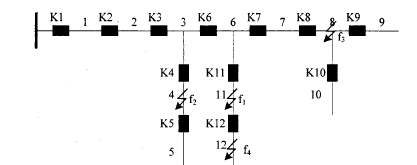一、故障信息的数学表示
在上图中K表示断路器，每一个断路器上均有一个FTU装置，可以反馈断路器开关是否过流，用表示上传的故障信息，反映的是各分段开关处是否流过故障电流有故障电流为1,否则为0）。即：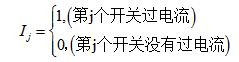因为FTU上传的信息可分为有故障信息及无故障信息两类,对于分段区间来讲也只能是有故障及无故障两种情况,所以我们可以用二进制编码规则对配电网故障定位问题进行数学建模。以上图所示辐射状配电网为例,系统拥有12个分段开关,我们可以用一串12位的二进制代码表示FTU的上传信息,作为程序的输入,1代表对应的开关有过流信息,0代表对应的开关无过流信息。同时用另一串12位的二进制代码作为程序的输出,代表对应馈线区间发生故障,代表无故障。
二、二进制PSO算法的配电网故障定位原理
应用二进制PSO算法求解配电网故障定位问题,粒子的位置代表配电网中馈线区段的状态,粒子的维数代表配电网的馈线区段总数。每一馈线区段存在0和1两种状态,0表示正常状态,1表示故障状态,馈线区段的状态为待求量。因此,N段馈线区段的状态求解就转化成N维粒子群优化求解,每个粒子的N维位置都表示为配电网N段馈线区段的潜在状态。每次迭代过程中,通过评价函数评价各粒子位置优劣,更新粒子的当前最优位置和全体粒子的最优位置,进而更新粒子的速度和位置,直到满足程序终止条件为止。最终得出的粒子群的全局最优位置就是所求的各馈线区段的实际状态。
三、构造配电网故障定位评价函数
基于待求各馈线区段实际状态下所对应的信息应与实际上传故障信息偏差最小的原则，构造如下评价函数：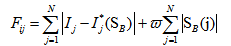表达式的值为每个潜在解对应的适应度值,值越小表示解越优良,因此评价函数应取极小值。
式中：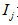为第j个开关FTU上传的故障信息,取值为1认为该开关流过了故障电流,为0则未流过；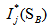为各开关节点的期望状态,若该开关流过了故障电流,则期望状态为1,相反,期望状态为0,表达式为各区段状态的函数。N为配电网中馈线区段的总数，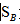为配电网中各设备状态,为1表明设备故障,取0则设备正常。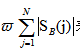表示一个权系数乘以故障设备数,。是根据故障诊断理论中“最小集”概念设置的权重系数,取值范围介于与1之间,表明故障区间数越少解越优,避免出现误诊断,本文中权系数取0.5。
四、求期望函数针对不同的网络，期望函数的表达式不同，无法用统一的格式表示出来。仅以上述算例为例来说明如何求取期望函数。
在辐射型配电网中,任何区间发生故障,必将导致其上游开关流过故障过电流。这是求取期望函数的原则。假设图一的馈线区段6处发生故障,那么分段开关K1,K2,K3 ,K6,都将有过电流若馈线区段11处发生故障,则K1,K2,K3 ,K6,K10都将有过电流。由此类推我们可得到各个分段开关的期望函数: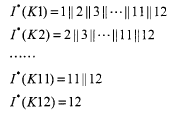用粒子群算法求解配电网故障定位评价函数的极小值即可。

Matlab源代码：

clc;
clear all;
global Ij;
global Vmax;                       %允许误差
MaxNum=120;                    %粒子最大迭代次数
D=12; %粒子的维数
Particlesize=30;                    %粒子群规模
c1=2.1;                            %每个粒子的个体学习因子，也称为加速常数
c2=2.1;                            %每个粒子的社会学习因子，也称为加速常数
w=1;                           %惯性因子
Vmax=4;                        %粒子的最大飞翔速度
S_b=randint(Particlesize,D);     %粒子所在的位置
V=Vmax*rand(Particlesize,D);         %粒子的飞翔速度
Ij=[1 1 1 1 0 1 1 1 0 0 1 1];%FTU 反馈的值这里就是输入，请修改这里，看结果
fitness=F(S_b');%这里注意下，要转置
%inline定义的适应度函数会使程序运行速度大大降低
for i=1:Particlesize
f(i)=F(S_b(i,:));
end
personalbest_x=S_b;%这里是种群里的每个粒子都认为自己是最好的
personalbest_faval=f;
[globalbest_favali]=min(personalbest_faval);%全局最优
globalbest_x=personalbest_x(i,:);
k=1;
Start=0;
while k<=MaxNum
Remember=globalbest_faval;
for i=1:Particlesize
f(i)=F(S_b(i,:));%计算适应度函数值
if f(i)<personalbest_faval(i) %判断当前位置是否是历史上最佳位置
personalbest_faval(i)=f(i);
personalbest_x(i,:)=S_b(i,:);
end
end%这里就是把整个种群中的所有粒子都检查了一遍，保证当前最优
[globalbest_favali]=min(personalbest_faval);%更新种群最优
globalbest_x=personalbest_x(i,:);
for i=1:Particlesize %更新粒子群里每个个体的最新位置
V(i,:)=w*V(i,:)+c1*rand*(personalbest_x(i,:)-S_b(i,:))...
+c2*rand*(globalbest_x-S_b(i,:));%更新了速度
for j=1:length(Ij)
if rand(1)<Sigmoid(V(i,j))%这里体现了速度决定位置
S_b(i,j)=1;
else
S_b(i,j)=0;
end
end
end
if globalbest_faval-Remember==0
Start=Start+1;
if Start==20
disp(globalbest_x);
break;
end
end
k=k+1;
end
F.m
function fitness=F(SB)
global Ij;
IE=zeros(1,length(Ij));
IE(1)=SB(1)|SB(2)|SB(3)|SB(4)|SB(5)|SB(6)|SB(7)|SB(8)|SB(9)|SB(10)|SB(11)|SB(12);
IE(2)=SB(2)|SB(3)|SB(4)|SB(5)|SB(6)|SB(7)|SB(8)|SB(9)|SB(10)|SB(11)|SB(12);
IE(3)=SB(3)|SB(4)|SB(5)|SB(6)|SB(7)|SB(8)|SB(9)|SB(10)|SB(11)|SB(12);
IE(4)=SB(4)|SB(5);
IE(5)=SB(5);
IE(6)=SB(6)|SB(7)|SB(8)|SB(9)|SB(10)|SB(11)|SB(12);
IE(7)=SB(7)|SB(8)|SB(9)|SB(10);
IE(8)=SB(8)|SB(9)|SB(10);
IE(9)=SB(9);
IE(10)=SB(10);
IE(11)=SB(11)|SB(12);
IE(12)=SB(12);
fitness=sum(abs(Ij-IE))+0.5*sum(abs(SB));
end
Sigmoid.m
function AN=Sigmoid(V)
global Vmax;
if V>Vmax
AN=0.98;
elseif V>-Vmax
AN=1/(1+exp(-V));
else
AN=-0.98;
end
end


展开全文• 在本例中，我们可以把x看作是粒子，函数值f(x)看作是适应度值（即粒子到最优对象的距离），适应度值越大（即粒子到最优对象越小）， 最大的适应度就是我们要求的最大值。 # -*- coding: utf-8 -*- import numpy ...
优化对象：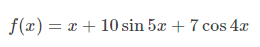求该函数在[-10, 10]区间的最大值。下面是该函数图像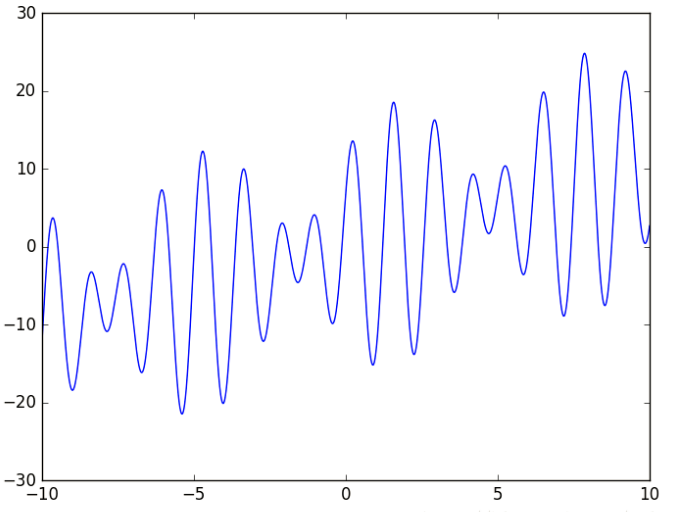在本例中，我们可以把x看作是粒子，函数值f(x)看作是适应度值（即粒子到最优对象的距离），适应度值越大（即粒子到最优对象越小）， 最大的适应度就是我们要求的最大值。

# -*- coding: utf-8 -*-

import numpy as np

# 粒子（鸟）
class particle:
def __init__(self):
self.pos = 0  # 粒子当前位置
self.speed = 0
self.pbest = 0  # 粒子历史最好位置

class PSO:
def __init__(self):
self.w = 0.5  # 惯性因子
self.c1 = 1  # 自我认知学习因子
self.c2 = 1  # 社会认知学习因子
self.gbest = 0  # 种群当前最好位置
self.N = 20  # 种群中粒子数量
self.POP = []  # 种群
self.iter_N = 100  # 迭代次数

# 适应度值计算函数
def fitness(self, x):
return x + 10 * np.sin(5 * x) + 7 * np.cos(4 * x)

# 找到全局最优解
def g_best(self, pop):
for bird in pop:
if bird.fitness > self.fitness(self.gbest):
self.gbest = bird.pos

# 初始化种群
def initPopulation(self, pop, N):
for i in range(N):
bird = particle()
bird.pos = np.random.uniform(-10, 10)
bird.fitness = self.fitness(bird.pos)
bird.pbest = bird.fitness
pop.append(bird)

# 找到种群中的最优位置
self.g_best(pop)

# 更新速度和位置
def update(self, pop):
for bird in pop:
# 速度更新
speed = self.w * bird.speed + self.c1 * np.random.random() * (
bird.pbest - bird.pos) + self.c2 * np.random.random() * (
self.gbest - bird.pos)

# 位置更新
pos = bird.pos + speed

if -10 < pos < 10: # 必须在搜索空间内
bird.pos = pos
bird.speed = speed
# 更新适应度
bird.fitness = self.fitness(bird.pos)

# 是否需要更新本粒子历史最好位置
if bird.fitness > self.fitness(bird.pbest):
bird.pbest = bird.pos

# 最终执行
def implement(self):
# 初始化种群
self.initPopulation(self.POP, self.N)

# 迭代
for i in range(self.iter_N):
# 更新速度和位置
self.update(self.POP)
# 更新种群中最好位置
self.g_best(self.POP)

pso = PSO()
pso.implement()

for ind in pso.POP:
print("x=", ind.pos, "f(x)=", ind.fitness)


程序执行中生成的种群结果：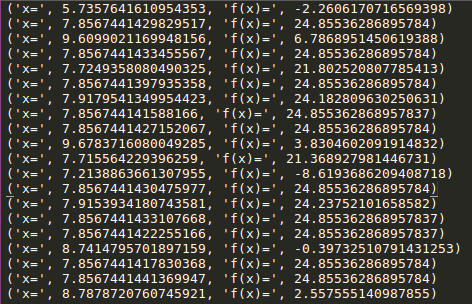得到 最优解为：

x=7.85674414          f（x）= 24.855362869

下面是绘图代码和代码执行结果：

# 绘图代码
import matplotlib.pyplot as plt
def func(x):
return x + 10 * np.sin(5 * x) + 7 * np.cos(4 * x)

x = np.linspace(-10, 10, 10000)
y = func(x)

scatter_x = np.array([ind.pos for ind in pso.POP])
scatter_y = np.array([ind.fitness for ind in pso.POP])
plt.plot(x, y)
plt.scatter(scatter_x, scatter_y, c='r')
plt.show()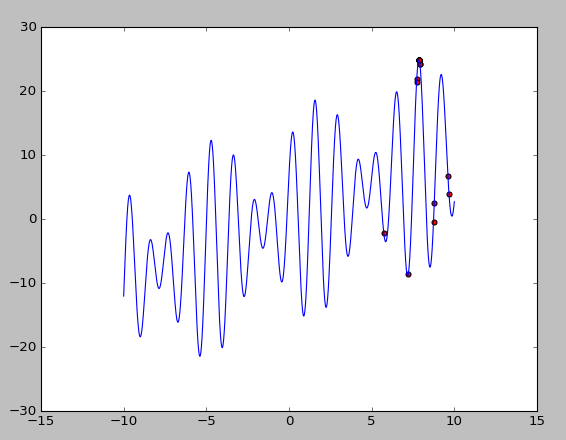展开全文• 10] # 粒子群取值范围（解空间范围） self.dim = 2 # 维度 # 初始化粒子群位置 self.x = np.random.uniform(low=self.boundary, high=self.boundary, size=(max_size, self.dim)) # max_num行，2列 # 初始化...
代码摘自：

https://blog.csdn.net/winycg/article/details/79120154

思路参考自：

https://zhuanlan.zhihu.com/p/107485342

原文采用的是固定权值，此处采用线性递减的权值策略，以提供另一种思路

'''
参考：
https://blog.csdn.net/winycg/article/details/79120154
https://zhuanlan.zhihu.com/p/107485342
原文采用的是固定权值，此处采用线性递减的权值策略，以提供另一种思路
'''
import numpy as np
import matplotlib.pyplot as plt
import time

class PSO:
def __init__(self, max_steps, max_size):

self.max_steps = max_steps  # 最大迭代次数
self.max_size = max_size  # 粒子数量
self.init_weight = self.cur_weight = 0.6  # 初始惯性权重与当前惯性权重
self.end_weight = 0.1
self.c1 = self.c2 = 2  # 个体学习因子、社会学习因子
self.boundary = [-10, 10]  # 粒子群取值范围（解空间范围）
self.dim = 2   # 维度
# 初始化粒子群位置
self.x = np.random.uniform(low=self.boundary,
high=self.boundary,
size=(max_size, self.dim)) # max_num行，2列
# 初始化粒子群速度
self.v = np.random.rand(self.max_size, self.dim)
# 计算当前所有粒子的适应值向量，所有粒子历史最佳适应度及其对应位置
self.curFitness = self.get_fitness(self.x)
self.individualBestFitness = self.curFitness
self.pbest = self.x
# 获取全局最佳适应度及其最佳位置
self.globalBestFitness = np.min(self.individualBestFitness)
self.gbest = self.x[np.argmin(self.individualBestFitness)] # np.argmin 给出水平方向最小值的下标

def get_fitness(self, x):
# 按行求平方和，即 X^2 + Y^2
return np.sum(np.square(x), axis=1)

def evolve(self):
self.iter = 0
for step in range(self.max_steps):
self.iter = self.iter + 1
self.cur_weight = (self.init_weight - self.end_weight) * float(self.max_steps - self.iter) / float(self.max_steps) + self.end_weight
# 生成两个随机数，分别代表飞向当前粒子历史最佳位置、全局历史最佳位置的程度
r1 = np.random.rand(self.max_size, self.dim)
r2 = np.random.rand(self.max_size, self.dim)
# 更新速度和权重
self.v = self.cur_weight * self.v + self.c1 * r1 * (self.pbest - self.x) \
+ self.c2 * r2 * (self.gbest - self.x)
self.x = self.x + self.v

# 画图
plt.clf()  # 清除当前 figure, 不关闭窗口
plt.scatter(self.x[:, 0], self.x[:, 1], s=30, color='r')  # s代表大小，k代表black
# x、y轴作图范围
plt.xlim(self.boundary, self.boundary)
plt.ylim(self.boundary, self.boundary)
# 画面停顿，以秒为单位
plt.pause(0.01)

# 计算当前所有粒子的适应值向量，所有粒子历史最佳适应度及其对应位置
self.curFitness = self.get_fitness(self.x)
update_id = np.greater(self.curFitness, self.individualBestFitness)
self.individualBestFitness[update_id] = self.curFitness[update_id]
self.pbest[update_id] = self.x[update_id]
# 获取全局最佳适应度及其最佳位置
if self.globalBestFitness > np.min(self.individualBestFitness):
self.globalBestFitness = np.min(self.individualBestFitness)
self.gbest = self.x[np.argmin(self.individualBestFitness)]
# 增加一个终止条件，以便计算寻找到最优值的耗时与迭代次数
if self.globalBestFitness - 0.0 < 1e-10:
break
print('global best fitness: %.5f, current mean fitness: %.5f' % (self.globalBestFitness, np.mean(self.curFitness)), end=" ")
print("最优位置：", self.gbest)

if __name__ == "__main__":

pso = PSO(100, 100)
start = time.time()
pso.evolve()
print("寻找到近似最优解时，共迭代了", pso.iter, "次,总共耗费了", (time.time() - start), "秒")
plt.show()



展开全文python3
• 遗传算法简单实例： matlab ...粒子群算法简单实例： matlab https://github.com/Zoyeeee/Particle-swarm-optimization-PSO- 蚁群算法简单实例： matlab https://github.com/Zoyeeee/ASO-
• 本文主要包含以下内容： 遗传算法和粒子群算法的程序设计的一般结构。主要介绍两类算法的程序设计中的主要思想。 介绍在Matlab编程中一些需要注意的细节。...一个遗传算法实例和一个粒子群算法实例
• matlab 粒子群算法实例，改程序就一个简单的小例子，阐述了粒子群优化算法的构造过程
•python 人工智能
• end end % 便利整个种群，求整个种群最佳的适应度和位置 %% 粒子群迭代 % 更新速度并对速度进行边界处理 % 更新位置并对位置进行边界处理 % 计算新种群各个个体位置的适应度 % 新适应度与个体历史最佳适应度做比较 ...matlab
• 数学建模源码集锦-基于混合粒子群算法的TSP搜索算法应用实例
• 数学建模源码集锦-基于粒子群算法的多目标搜索算法应用实例
• 数学建模源码集锦-基于动态粒子群算法的动态环境寻优算法应用实例
• 1、基本粒子群算法 假设在一个 D 维的目标搜索空间中，有 N 个粒子组成一个群落，其中第i 个 粒子表示为一个 D 维的向量： $$X_i=(x_{i1},x_{i2}, \cdots ,x_{iD}), \quad i=1,2, \cdots ,N \quad \text {(1)}$$...算法 matlab
• 不仅提供了粒子群算法代码，还提供了10维测试函数实例
• 多个函数利用多种粒子群算法解决优化问题： 用二阶粒子群优化算法求解无约束优化问题 用二阶振荡粒子群优化算法求解无约束优化问题 用混沌粒子群优化算法求解无约束优化问题 用基于选择的粒子群优化算法求解无...
• 今天给大家讲解的时粒子群算法，首先先牢记以下的基本公式： 1.简单的来讲，粒子群算法是这个样子，当你在一个全解的范围内，想要去找最优解，可以先派出像四面八方而去的小兵去搜索，他们向四面八方去探索时在坚守...
• 使用matlab编程，分为多个.m文件编写，包括支配关系选择，全局领导者选择，删除多于的非劣解，创建...套用算法只需要改动Mycost函数与主函数中粒子的取值与维度，即主函数中问题定义处与MOPSO设置处，其余不需要改动
• 视频讲解+粒子群优化算法PPT+演讲稿+matlab算法实例...